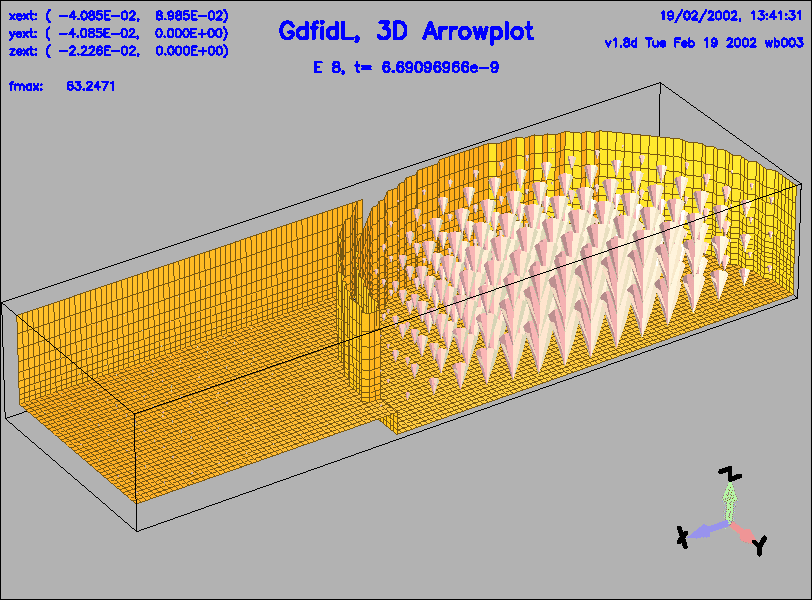# How to compute the External Q of a loaded Cavity

The computation of the external Q of a cavity with an attached waveguide is via three simple steps.

• Computation of the wanted resonant field
• Computation of the ringing of the resonant field in a geometry with an attched waveguide
• Analysis of the power flowing with the postprocessor

# First step, computation of resonant fields

In the first step, we compute the resonant fields in a closed geometry, ie. the waveguide is closed with an electric or magnetic boundary condition.

    gd1 < cavity-with-waveguide.gdf | tee out-eigen


After some minutes we get a list of frequencies like:

  One solution seems to be static and is not saved.
i   freq(i)       acc(i)         cont(i)
1   27.6778e+6   0.9982920236  0.3328254536       # "grep" for me
2    2.8818e+9   0.0000000790  0.0000000695       # "grep" for me
3    3.6905e+9   0.0000000162  0.0000000313       # "grep" for me
4    4.5837e+9   0.0000000003  0.0000000005       # "grep" for me
5    6.1361e+9   0.0000000103  0.0000000206       # "grep" for me
6    6.4118e+9   0.0000000013  0.0000000151       # "grep" for me
7    6.6111e+9   0.0000000084  0.0000000998       # "grep" for me
8    6.8857e+9   0.0000000062  0.0000000463       # "grep" for me
9    7.3426e+9   0.0000000095  0.0000000774       # "grep" for me
10    7.5733e+9   0.0000005696  0.0000093463       # "grep" for me
11    7.6269e+9   0.0000000112  0.0000001527       # "grep" for me
12    7.9060e+9   0.0000000082  0.0000000684       # "grep" for me
13    8.3725e+9   0.0013724901  0.0123083161       # "grep" for me
14    8.4666e+9   0.0060409112  0.2701361389       # "grep" for me

The inputfile for this cavity is cavity-with-waveguide.gdf

# Second step

We load the resonant field with the right frequency ( 2.88 GHz ) into the computational volume and let it ring. This time, we apply an absorbing boundary condition where the waveguide crosses the computational volume. We record the power flowing through that waveguide.
     gd1 < let-it-ring.gdf | tee out-fdtd

The inputfile for this time-domain computation is let-it-ring.gdf

# Third and last step

We use the postprocessor to look at the power flowing through the waveguide, and to compute the stored energy at a selected time. Since the cavity is only sligthly coupled, the power flowing is almost constant ( the external Q is very high ).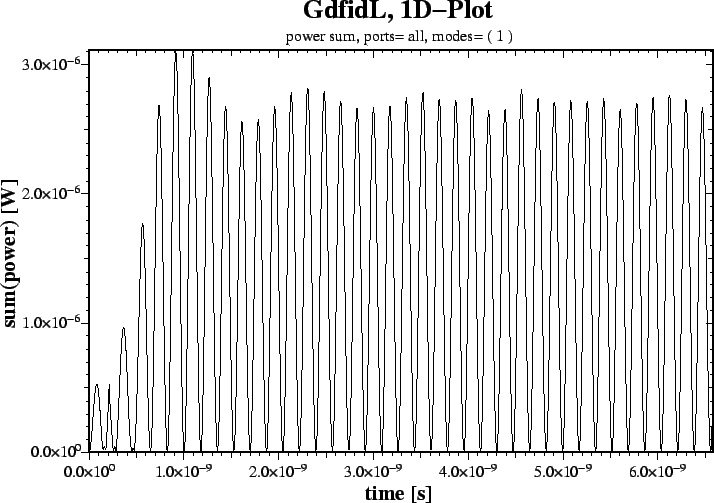We start the postprocessor, gd1.pp, and use him interactively:
Input for gd1.pp:

     -general, infile= @last

-sparameter
freqdata= no
timedata= yes
tsumpower= yes
doit

We get a lot of plots. One of these plots shows us the power sum of all selected modes as a function of time. ..But only one mode is selected.. After about 20 periods, the transients have decayed sufficiently, leaving only a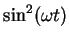function, which is the power as a function of time.
The average power is about 1.4e-6 Watts.

In order to know the external Q, we have to know what the stored energy is. Since the power flowing is almost constant, the stored energy will also be almost constant...

We compute the stored energy in the electric and magnetic field with the section "-energy" of gd1.pp:

    -energy
solution= 8
quantity= e, doit
quantity= h, doit

 ##############################################################################
# Flags: nomenu, prompt, message,                                            #
##############################################################################
# section: -energy                                                           #
##############################################################################
# symbol   = h_8                                                             #
# quantity = h                                                               #
# solution = 8                                                               #
#                                                                            #
#                                                                            #
# @henergy : 4.88692e-12               (symbol: h_8, m: 1)                   #
# @eenergy : 253.6925e-15              (symbol: e_8, m: 1)                   #
##############################################################################
# doit, ?, return, end, help                                                 #
##############################################################################

We see that at the time when the 8.th field was stored ( t=6.6901e-9 s ), the total stored energy in the computational volume was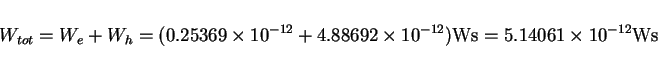(1)

The external Q for the chosen aperture width therefore is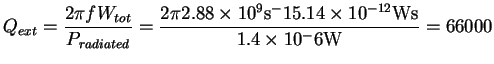(2)

 -------
Thats it.

Although not necessary, we want to have a look at the electric field after about 20 periods: